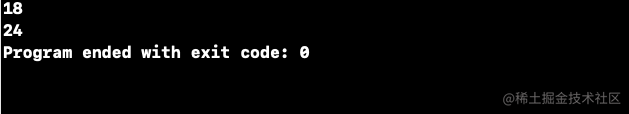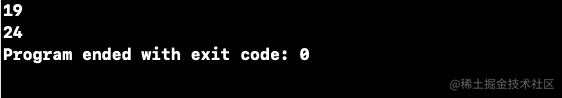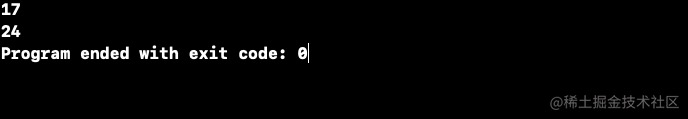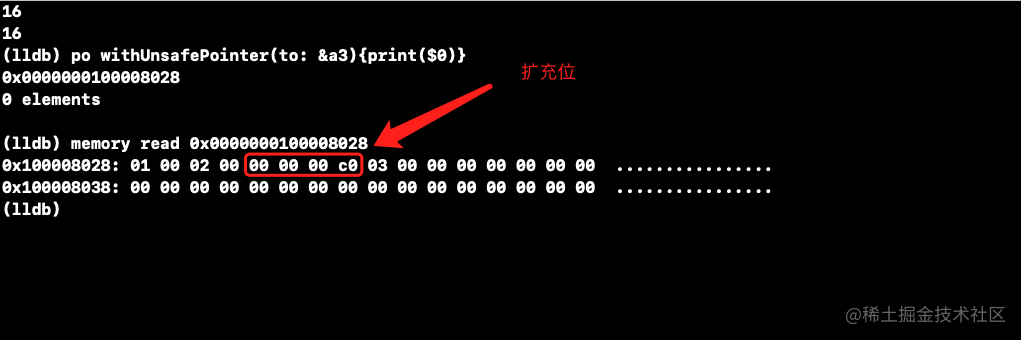# 关联枚举的内存布局(一)

``````enum A {
case a0(a:Int8) //这里是Int8类型
case a1(a:Int8,b:Int,c:Int16)
}
print(MemoryLayout<A>.size)
print(MemoryLayout<A>.stride)``````enum A {
case a0(a:Int) //这里是Int类型，在64位系统下占8字节
case a1(a:Int8,b:Int,c:Int16)
}
print(MemoryLayout<A>.size)
print(MemoryLayout<A>.stride)``````enum Test{
case enum1(t1:Int)
case enum2(t1:Int8,t2:Int16,t3:Int)
case enum3(t1:Int8,t2:Int,t3:Int16,t4:Int32)
case enum4(t1:Int8,t2:Int,t3:Int16,t4:Int,t5:Int8,t6:String)
}

enum1为8字节不需要对齐 占用8

enum2占用(1,2,8)共11字节,因为1除以2无法整除则需要把1变为2占用则变为(2,2,8),又因为2+2除以8不能整除则需要补4即把2变为6占用为(2,6,8)

enum3占用(1,8,2,4)共15字节 因为1除以8无法整除需要补7 变为(8,8,2,4),此时8+8除以2整除故不变,继续往后看8+8+2无法整除4 需要补2 变为(8,8,4,4)

enum4特殊一点 (1,8,2,8,1,16) 因为1无法整除8故需要补7 (8,8,2,8,1,16) 因为8+8整除2故不变 (8,8,2,8,1,16) 因为8+8+2不能整除8 故补6 (8,8,8,8,1,16) 因为8+8+8+8整除1 故不变 (8,8,8,8,1,16) 因为8+8+8+8+1=33,这时用33和谁比较呢,我们知道系统都是8字节对齐的,扩充16字节势必会浪费空间,我们继续和8比较 需要补7 (8,8,8,8,8,16)

``````enum A {
//1
case a0(a:Int8)

// 1+1=2
case a1(a:Int8,b:Int8)

//1+2+8，1/2不为正整数->2+2+8 4/8不为正整数->2+6+8=16
case a2(a:Int8,b:Int16,c:Int)

// 1+4+8 1/4不为正整数->4+4+8 8/8为正整数->4+4+8=16
case a3(a:Int8,b:Int32,c:Int)
}

//1-8字节只有一个Int8类型的值，是1字节对齐
//1-8字节内存布局 01 00 00 00 00 00 00 00
var a0 = A.a0(a: 1)

//1-8字节存储了两个Int8类型的值，是1字节对齐
//1-8字节内存布局 01 02 00 00 00 00 00 00
var a1 = A.a1(a: 1, b: 2)

//1-8字节存储了一个Int8类型的值一个Int16类型的值，是2字节对齐
//1-8字节内存布局 01 00 02 00 00 00 00 00
var a2 = A.a2(a: 1, b: 2,c: 3)

//1-8字节存储了一个Int8类型的值一个Int32类型的值，是4字节对齐
//1-8字节内存布局 01 00 00 00 02 00 00 00
var a3 = A.a3(a: 1, b: 2,c: 3)

print(MemoryLayout<A>.size)
print(MemoryLayout<A>.stride)
print("end")

a0真实存储位：

a0扩充位：[2,3,4,5,6,7,8,9,10,11,12,13,14,15,16]

a1真实存储位：[1,2]

a1扩充位：[3,4,5,6,7,8,9,10,11,12,13,14,15,16]

a2真实存储位：[1,3,4,9,10,11,12,13,14,15,16]

a2扩充位：[2,5,6,7,8]

a3真实存储位：[1,5,6,7,8,9,10,11,12,13,14,15,16]

a3扩充位：[2,3,4]

``````enum A {
case a0(a:Int8) //1字节
case a1(a:Int8,b:Int8) //1+1=2字节
case a2(a:Int8,b:Int16,c:Int) //8+8=16字节
case a3(a:Int8,b:Int32,c:Int) //8+8=16字节
}

//size应该是16+1=17字节
print(MemoryLayout<A>.size)
print(MemoryLayout<A>.stride)``````enum A {
//1
case a0(a:Int8)

// 1+1=2
case a1(a:Int8,b:Int8)

//1+2+8，1/2不为正整数->2+2+8 4/8不为正整数->2+6+8=16
case a2(a:Int8,b:Int16,c:Int)

//1+2+8，1/2不为正整数->2+2+8 4/8不为正整数->2+6+8=16
case a3(a:Int8,b:Int16,c:Int)
}

//1-8字节只有一个Int8类型的值，是1字节对齐
//1-8字节内存布局 01 00 00 00 00 00 00 00
var a0 = A.a0(a: 1)

//1-8字节存储了两个Int8类型的值，是1字节对齐
//1-8字节内存布局 01 02 00 00 00 00 00 00
var a1 = A.a1(a: 1, b: 2)

//1-8字节存储了一个Int8类型的值一个Int16类型的值，是2字节对齐
//1-8字节内存布局 01 00 02 00 00 00 00 00
var a2 = A.a2(a: 1, b: 2,c: 3)

//1-8字节存储了一个Int8类型的值一个Int32类型的值，是4字节对齐
//1-8字节内存布局 01 00 00 00 02 00 00 00
var a3 = A.a3(a: 1, b: 2,c: 3)

print(MemoryLayout<A>.size)
print(MemoryLayout<A>.stride)
print("end")

a0、a1、a2、a3的真实存储位扩充位

a0真实存储位：

a0扩充位：[2,3,4,5,6,7,8,9,10,11,12,13,14,15,16]

a1真实存储位：[1,2]

a1扩充位：[3,4,5,6,7,8,9,10,11,12,13,14,15,16]

a2真实存储位：[1,3,4,9,10,11,12,13,14,15,16]

a2扩充位：[2,5,6,7,8]

a3真实存储位：[1,3,4,9,10,11,12,13,14,15,16]

a3扩充位：[2,5,6,7,8]

``````enum A {
case a0(a:Int8) //1字节
case a1(a:Int8,b:Int8) //1+1=2字节
case a2(a:Int8,b:Int16,c:Int) //8+8=16字节
case a3(a:Int8,b:Int16,c:Int) //8+8=16字节
}

var a3 = A.a3(a: 1, b: 2, c: 3)
print(MemoryLayout<A>.size)
print(MemoryLayout<A>.stride)
print("end")iOS

iOS